# Lens¶

SIMION has often been used to simulate 2D and 3D lens systems, either in isolation or as components of other systems. The three-element Einzel lens example in SIMION is demonstrated in A Tour of the SIMION Demo.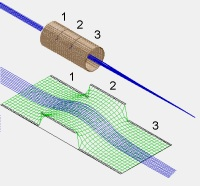Fig. 36 Figure: SIMION Einzel lens example (above) with corresponding potential energy surface (below).

Some papers covering the simulations of lenses in SIMION include

## Theory¶

### Helmholtz-Lagrange Law¶

The Helmholtz-Lagrange Law relates the linear magnification M and angular magnification m of rays through an electrostatic lens to the ratio of potentials between the two ends of the lens. Linear magnification is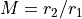, where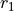and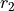are the displacements from the axis of the object and image locations. Angular magnification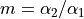, where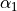and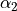are the pencil angles of the object and image points. The pencil angle is the half angle of the beam.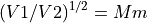See the SIMION Example: lens_properties for an example of Helmholtz-Lagrange Law.

### Snell’s Law¶

See Light Optics and SIMION Example: lens_properties for a demonstration of Snell’s law.

### Cardinal Points¶

Carinal points describe a lens. The symbols as commonly used by Heddle and elsewhere are given below.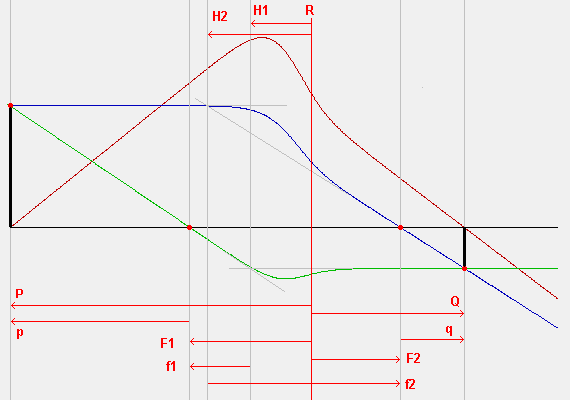Fig. 37 Figure: Cardinal points in a lens, demonstrated with SIMION particle traces in SIMION Example: lens_properties

• R - reference plane. The location of this plane is arbitrary, but it is usually, by convention, taken as the center of the center lens and defined as x=0.
• P - object position. This is the location of the object being imaged. It is relative to R. The principle rays intersect here.
• Q - image position. This is the location of the image of the object P. It is relative to R. The principle rays intersect here too.
• F1 - first focal point (mid-focal distance). This is the location of the intersection of second principle ray and axis. It is relative to R.
• F2 - second focal point (mid-focal distance). This is the location of the intersection of the first principle and axis. It is relative to R.
• H1 - first principle plane. This is the location of the intersection of the second principle ray asymptotes. One of the asympotes may be approximated as the tangent of the ray through F1 (assuming the lens is not too strong).
• H2 - second principle plane. This is the location of the intersection of the first principle ray asymptotes. One of the asympotes may be approximated as the tangent of the ray through F2 (assuming the lens is not too strong).
• p - This is the distance of P relative to F1.
• q - This is the distance of Q relative to F2.

## Screenshots¶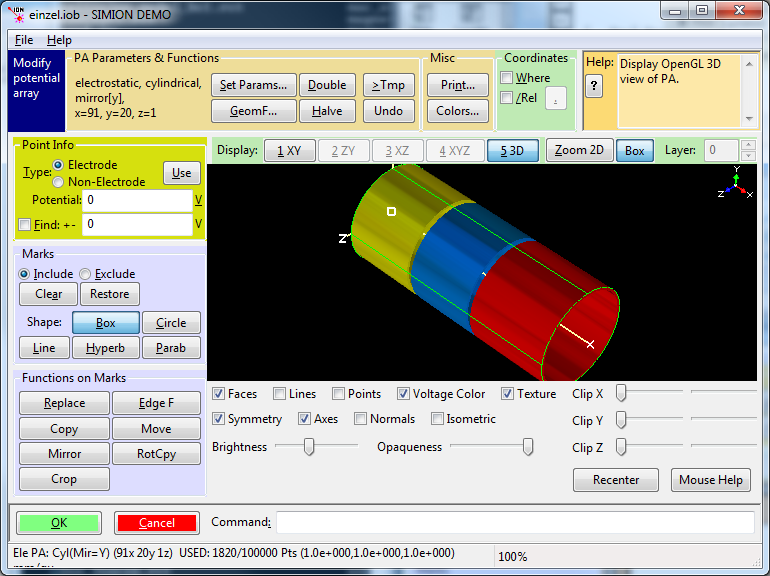Einzel lens geometry (three cylindrical lenses), from SIMION Example: einzel.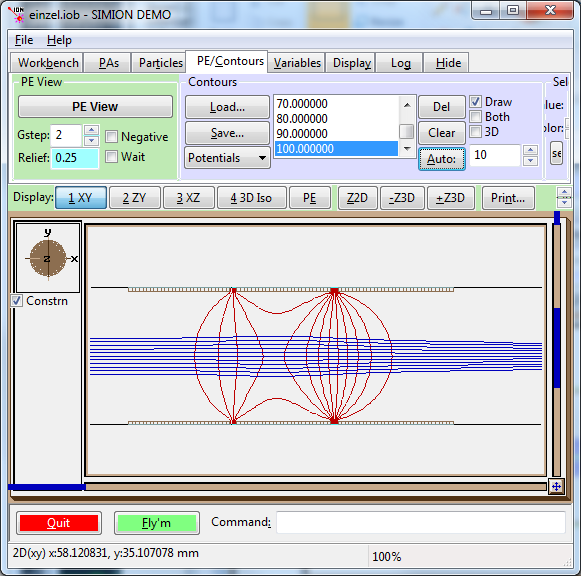Equipotential lines showing electric field.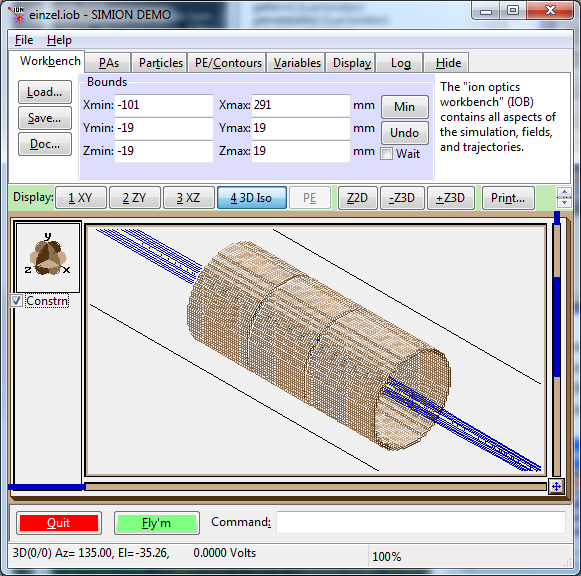Particles flying through lens.